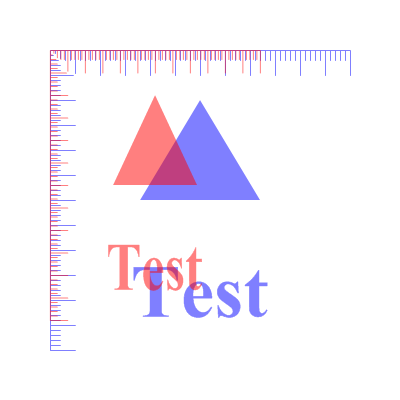ScaleCoordinates()

Syntax

ScaleCoordinates(ScaleX.d, ScaleY.d [, System])
Description
Scale the vector drawing coordinate system by stretching it in the x/y direction.

Parameters

 ScaleX.d, ScaleY.d The scale factor for each direction. A factor of 1.0 leaves the coordinates unchanged while factors above and below 1.0 stretch the coordinate system. A negative factor mirrors the output corrordinates. System (optional) Specifies the coordinate system to change. This can be one of the following values: ``` #PB_Coordinate_User : Change the coordinate system for points in the drawing path (default) #PB_Coordinate_Source: Change the coordinate system for the vector drawing source ```

Return value

None.

Remarks

See the vectordrawing overview for an introduction to the different coordinate systems.

The following image demonstrates the effect of scaled coordinates. The same figure is drawn twice, the original is in blue, and the version with scaled coordinates is in red.Example

```  If OpenWindow(0, 0, 0, 400, 200, "VectorDrawing", #PB_Window_SystemMenu | #PB_Window_ScreenCentered)
CanvasGadget(0, 0, 0, 400, 200)

If StartVectorDrawing(CanvasVectorOutput(0))
VectorFont(LoadFont(0, "Times New Roman", 60, #PB_Font_Bold))

VectorSourceColor(RGBA(0, 0, 255, 128))
MovePathCursor(50, 50)
DrawVectorText("Test")

ScaleCoordinates(0.7, 0.9)

VectorSourceColor(RGBA(255, 0, 0, 128))
MovePathCursor(50, 50)
DrawVectorText("Test")

StopVectorDrawing()
EndIf

Repeat
Event = WaitWindowEvent()
Until Event = #PB_Event_CloseWindow
EndIf
```See Also

ResetCoordinates(), TranslateCoordinates(), RotateCoordinates(), SkewCoordinates(), FlipCoordinatesX(), FlipCoordinatesY(), ConvertCoordinateX(), ConvertCoordinateY()

Supported OS

All

<- SaveVectorState() - VectorDrawing Index - SkewCoordinates() ->